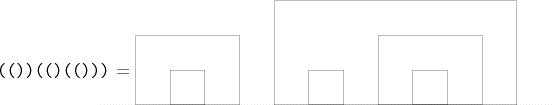# Internet Problem Solving Contest

## Problem E – Easy representation

Peter is preparing slides for his lecture on parsing arithmetic expressions. In the first part of the lecture he wants to focus just on parsing brackets. He invented an interesting geometric representation of a correct bracket sequence for his students, because one image is better than a thousand words:Formally, the definition of the geometric representation looks as follows. The simplest correct bracket sequence () is represented by a 1 × 1 square. If A is a correct bracket sequence and g(A) its represenation, then the representation for (A) is g(A) surrounded by a rectangle two units wider than g(A) and one unit taller than the highest point of g(A). If A and B are two correct bracket sequences and g(A) and g(B) are their representations, then we get g(AB) by placing g(B) one unit to the right of g(A).

After he finished his slides, Peter started to play with the images he prepared. He painted the bounded areas of the images alternately black and white, in such a way that the outer-most areas are all painted black. For the example above this coloring looks as follows:Problem specification

You are given a correct bracket sequence. Calculate the area that is colored black.

Input specification

The first line of the input file contains an integer T specifying the number of test cases. Each test case is preceded by a blank line.

Each test case consists of one line with a correct bracket sequence. Every line will only contain characters ‘(’ and ‘)’.

Output specification

For each test case output one line with one integer – the area of the black part of the corresponding geometric representation.

Example

 input 2    ((()))    (())(()(()))
 output 10  20 The second test case is the one shown in the pictures above.